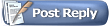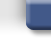Project64 Forums'nother SP problem: with VCR
 FAQ Members List Calendar Search Today's Posts Mark Forums Read#1HatCatAlpha Tester Project Supporter Senior Member Join Date: Feb 2007 Location: In my hat. Posts: 16,236'nother SP problem: with VCR

Code:
```void RSP_Vector_VCR (void) {
int count, el, del;

RSP_Flags.UW = 0;
RSP_Flags.UW = 0;
RSP_Flags.UW = 0;
for (count = 0;count < 8; count++) {
el = Indx[RSPOpC.rs].B[count];
del = EleSpec[RSPOpC.rs].B[el];

if ((RSP_Vect[RSPOpC.rd].HW[el] ^ RSP_Vect[RSPOpC.rt].HW[del]) < 0) {
if (RSP_Vect[RSPOpC.rt].HW[del] < 0) {
RSP_Flags.UW |= ( 1 << (15 - el));
}
if (RSP_Vect[RSPOpC.rd].HW[el] + RSP_Vect[RSPOpC.rt].HW[del] <= 0)
{
RSP_ACCUM[el].HW = ~RSP_Vect[RSPOpC.rt].UHW[del];
RSP_Flags.UW |= ( 1 << (7 - el));
} else {
RSP_ACCUM[el].HW = RSP_Vect[RSPOpC.rd].HW[el];
}
} else {
if (RSP_Vect[RSPOpC.rt].HW[del] < 0) {
RSP_Flags.UW |= ( 1 << (7 - el));
}
if (RSP_Vect[RSPOpC.rd].HW[el] - RSP_Vect[RSPOpC.rt].HW[del] >= 0)
{
RSP_ACCUM[el].HW = RSP_Vect[RSPOpC.rt].UHW[del];
RSP_Flags.UW |= ( 1 << (15 - el));
} else {
RSP_ACCUM[el].HW = RSP_Vect[RSPOpC.rd].HW[el];
}
}
RSP_Vect[RSPOpC.sa].HW[el] = RSP_ACCUM[el].HW;
}
}```
Numerous standard references define this to be incorrect.

VCR is a single-precision operation wielding one's complement logic.
In place of the code I have highlighted red, the following complies to the correct operation.
Code:
```
if (RSP_Vect[RSPOpC.rd].HW[el] + RSP_Vect[RSPOpC.rt].HW[del] + 1 <= 0)```
It is only an issue if either `VR[vs][source] <= 0` or `VR[vt][target] <= 0`, but not both.
#2HatCatAlpha Tester Project Supporter Senior Member Join Date: Feb 2007 Location: In my hat. Posts: 16,236Actually there may be an alternative, faster way of correcting this.

`if (x + y + 1 <= 0)`

`if (x + y < 0)`

hmm...duh, we can.
Because:

x + y + 1 <= 0
x + y + 1 - 1 <= 0 - 1
x + y <= -1

In the C processor, x and y are both integers, never FP numbers.
We can therefore safely assume the next rewrite:

x + y < 0

It wasn't the standard algorithm, but it still accomplishes the exact same thing only faster in the code generation.

Umm either way zilmar's algorithm needs fixing so yea#3HatCatAlpha Tester Project Supporter Senior Member Join Date: Feb 2007 Location: In my hat. Posts: 16,236Yep, makes a difference.

If I check `if (x + y + 1 <= 0)`, Microsoft assembly output is as follows:
Code:
```; 14   :                 le = (VR[vs].s[i] + VR[vt].s[i] + 1 <= 0);

movsx	ecx, cx
mov	DWORD PTR tv5210[ebp], eax
xor	eax, eax
mov	esi, edx
movsx	edx, di
mov	DWORD PTR tv4351[ebp], edx
lea	edx, DWORD PTR [ecx+edx+1]
test	edx, edx
setle	al```
It couldn't figure out the simple math to optimize the inequality like I just did by writing `if (x + y < 0)`:
Code:
```; 14   :                 le = (VR[vs].s[i] + VR[vt].s[i] < 0);

movsx	ecx, cx
mov	DWORD PTR tv5180[ebp], eax
xor	eax, eax
mov	esi, edx
movsx	edx, di
mov	DWORD PTR tv4323[ebp], edx
sets	al```
Makes me wonder if MarathonMan can get GCC to figure it out for him. Part of me doesn't mind being confined to unintelligent compilers, since I like to do some of the scratch optimizations by hand, but to keep code readable I kind of have to assume the compiler wasn't made by Microsoft. T_T
#4MarathonManAlpha Tester Project Supporter Senior Member Join Date: Jan 2013 Posts: 454I don't even need to try that with gcc. I'll likely do better than that, too. `sets` isn't a fast instruction, since it depends on the condition code to execute.
#5HatCatAlpha Tester Project Supporter Senior Member Join Date: Feb 2007 Location: In my hat. Posts: 16,236Better/faster, possibly.
There is always that chance.

Though more accurate? I would have to doubt.The implementation that you may find in my `rsp\vu\vcr.h` opcode header is basically an identical mapping of the algorithm defined in the ***RTFM-that-must-not-be-named***, uh that got sold on that one episode of Hairy Pothead, nothing commercial/protected or anything like that. :/

In this instance, assume that element == 0x0.
Code:
```    if (element == 0x0) /* if (element >> 1 == 00) */
for (i = 0; i < 8; i++)
if ((VR[vs].s[i] ^ VR[vt].s[i]) < 0)
{
ge = (VR[vt].s[i] < 0);
le = (VR[vs].s[i] + VR[vt].s[i] < 0); /* vs + vt + 1 <= 0 */
VACC[i].s[LO] = le ? ~VR[vt].s[i] : VR[vs].s[i];
VCC |= (ge << (i + 8)) | (le << (i + 0));
}
else
{
le = (VR[vt].s[i] < 0);
ge = (VR[vs].s[i] - VR[vt].s[i] >= 0);
VACC[i].s[LO] = le ? VR[vt].s[i] : VR[vs].s[i];
VCC |= (ge << (i + 8)) | (le << (i + 0));
}```
That completes the entire wave of operation simulation for VCR.

If you can do better I am excited to see what you can come up with sometime. Not saying at all that I doubt you. I believe there may be a speed-up by violating the principle of the suggested algorithm in the default reference, for example by cutting the if/else split into a simple check on whether VR[vt].s[i] < 0 (used under both branch blocks).

Yes it depends on the condition code to execute, but the vector select clips have a shitload of conditions. Aside from caching all possible combinations of datuum into some huge lookup table I must admit I have no idea how you would eliminate the need for such methods.
#6MarathonManAlpha Tester Project Supporter Senior Member Join Date: Jan 2013 Posts: 454Quote:
 Originally Posted by FatCatBetter/faster, possibly. There is always that chance. Though more accurate? I would have to doubt.Heh. I don't understand the algorithm yet, but as long as it conforms to the standards, then gcc will generate correct code. No questions.Quote:
 If you can do better I am excited to see what you can come up with sometime. Not saying at all that I doubt you. I believe there may be a speed-up by violating the principle of the suggested algorithm in the default reference, for example by cutting the if/else split into a simple check on whether VR[vt].s[i] < 0 (used under both branch blocks). Yes it depends on the condition code to execute, but the vector select clips have a shitload of conditions. Aside from caching all possible combinations of datuum into some huge lookup table I must admit I have no idea how you would eliminate the need for such methods.
http://static.quickmeme.com/media/social/qm.gif

Need to do some regression testing on my VMADL and then I'll wrinkle my brain some more.
#7HatCatAlpha Tester Project Supporter Senior Member Join Date: Feb 2007 Location: In my hat. Posts: 16,236Also...it's like I keep forgetting, you use SSSE3 and SSE4 XD.
No way in hell could I do it faster without SSE than what you do.
I thought that went without saying.

But from a scalar perspective I think I have it optimized pretty well enough.

I wasn't doubting that GCC would generate the correct code.
In my experience and memories I am sure it would have, though I haven't really tested that yet. I figure, MSVC doesn't optimize it and forced me to do it, but GCC probably would have figured it out.

My confidence in that being one of the reasons why I have not tested.

Anyway, I hope you at least finished VMUDL first because that is extremely easy, like the easiest of the multiplies.
Makes doing VMADL a bit easier to figure out based on that right#8MarathonManAlpha Tester Project Supporter Senior Member Join Date: Jan 2013 Posts: 454Quote:
 Originally Posted by FatCatAnyway, I hope you at least finished VMUDL first because that is extremely easy, like the easiest of the multiplies. Makes doing VMADL a bit easier to figure out based on that rightVMADH was a joke in SSE. VMADL is actually the hardest on. The reason being is that SSE only has 32-bit addition functions, and I refuse to write any loops, so I have to process all 8 vectors at once and things get a little crazy.
#9HatCatAlpha Tester Project Supporter Senior Member Join Date: Feb 2007 Location: In my hat. Posts: 16,236The basic algorithm behind VMUDL and VMADL is the same, except multiply-add equals which you have already proven you are more than capable of integrating.

So if you are able to do VMUDL I have no idea why VMADL would be so much harder.

All I really had to do with VMADL was right-shift each 32-bit product (without sign-extension!) by 16 bits and presto! VR = VACC = result; ... no clue why VMADH would be so much easier, but VMUDL was my very first vector opcode to fully figure out cause it was so easy and small.#10MarathonManAlpha Tester Project Supporter Senior Member Join Date: Jan 2013 Posts: 454Oh, heh. I've only done the VMADs. I haven't done the VMUDs yet. Maybe I mis-spoke somewhere.Thread ToolsShow Printable VersionEmail this Page Display ModesLinear ModeSwitch to Hybrid ModeSwitch to Threaded ModePosting Rules You may not post new threads You may not post replies You may not post attachments You may not edit your posts BB code is On Smilies are On [IMG] code is On HTML code is Off
 Forum Jump User Control Panel Private Messages Subscriptions Who's Online Search Forums Forums Home General Discussion     Site News     Open Discussion Public Version     Project 64 - v2.x - Suggestions     Project 64 - v2.x - Issues     Project64 - Android     Project 64 - v1.6

All times are GMT. The time now is 08:31 PM.Contact Us - Archive - Top